# Basic Logic Gates Truth table

Electronic Logic Gates are used to implement Boolean functions practically. Electronic Logic Gates are comes in Integrated Circuits (IC) package. Almost every electronic logic gates requires Power supply to operate, gets input as Logic 0 and Logic 1 then gives output as Logic 0 and Logic 1.

here,

Logic 0 = LOW = OFF = 0V.

Logic 1 = HIGH = ON = 5V.

Logic gates are takes some time delay to produce output from input. Every Logic Gate operations are stated by its truth table, and it is like a input and corresponding output chart.

## Laws of Boolean Algebra

A . B = B . A

A + B = B + A

### Associative law

A + (B + C) = (A + B) + C = A + B + C

A(B.C) = (A.B)C = A . B . C

### Distributive law

A(B + C) = A.B + A.C

A + (B.C) = (A + B).(A + C)

## DeMorgan’s Theorems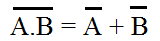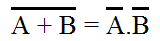A.0 = 0 A.1 = A

A.A = A A.Ā = 0

A+0 = A A+1 = 1

A+A = A A+Ā = 1

NOT A = Ā

NOT Ā = A

## AND gate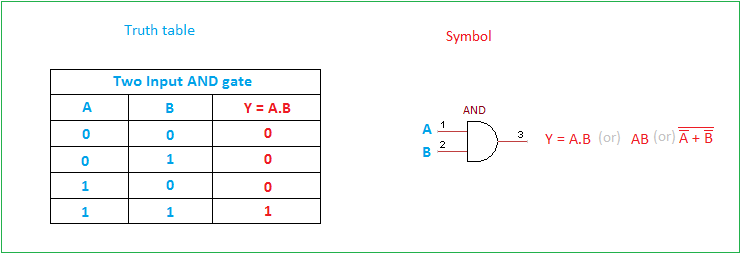AND gate performs Boolean multiplication between given inputs. It gives Logical High output only when all inputs are High.

## OR gate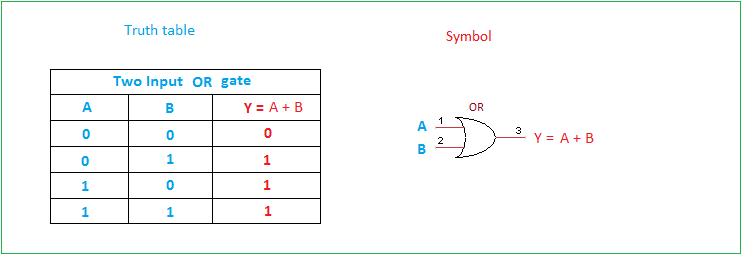OR gate performs Boolean Addition between given inputs. It gives Logical Low output only when all inputs are Low.

## NOT gate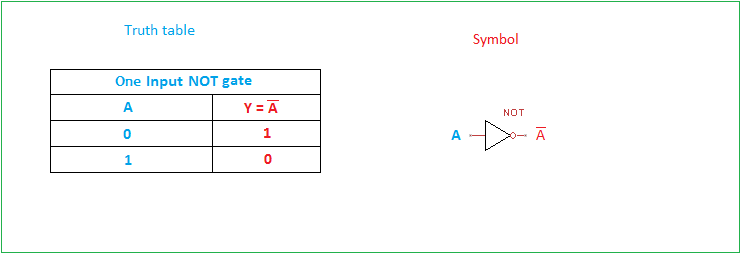NOT gate performs Boolean Invert or complement of given input, this NOT gate mostly invert single input some times it may in array form. When we give LOW input output of NOT gate will be HIGH and vice versa.

## NAND gate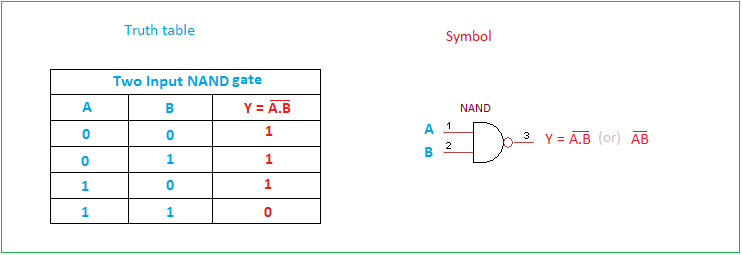NAND gate is a universal logic gate, this gate contains AND gate & NOT gate then gives logical LOW only when all inputs are High.

## NOR gate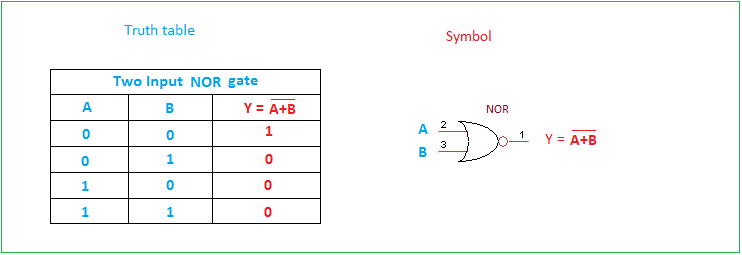NOR gate is universal logic gate, this gate contains OR gate & NOT gate then gives logical HIGH only when all inputs are Low.

## EXOR gate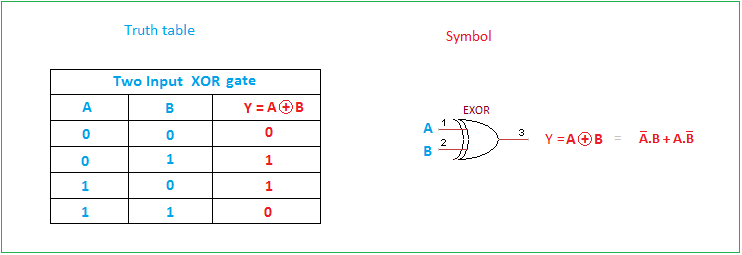Ex-OR gate may referred as Exclusive OR gate, it gives logical LOW output when both inputs are either Low or High.

## EXNOR gate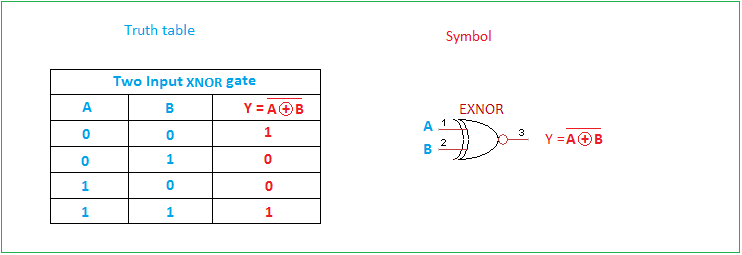EX-NOR gate may referred as Exclusive NOR gate, it give complement output of EXOR gate that is, it gives logical HIGH output when both inputs are High or both inputs are LOW.

Logic gates Examples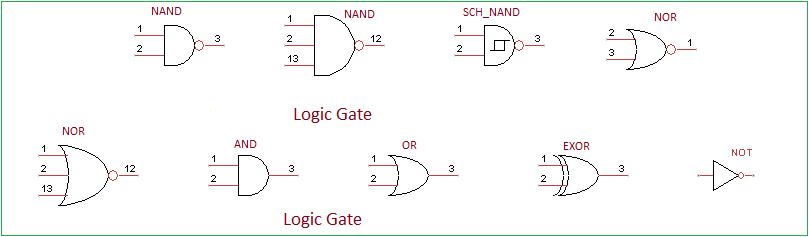Different kind of logic gates are available, like two input or three input or quad input logic gates. We  can implement these logic gates depends on our digital electronics design.